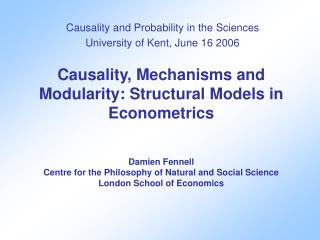# Causality and Probability in the Sciences University of Kent, June 16 2006 - PowerPoint PPT PresentationDownload PresentationCausality and Probability in the Sciences University of Kent, June 16 2006

Download Presentation## Causality and Probability in the Sciences University of Kent, June 16 2006

- - - - - - - - - - - - - - - - - - - - - - - - - - - E N D - - - - - - - - - - - - - - - - - - - - - - - - - - -
##### Presentation Transcript

1. Causality, Mechanisms and Modularity: Structural Models in EconometricsDamien FennellCentre for the Philosophy of Natural and Social ScienceLondon School of Economics Causality and Probability in the Sciences University of Kent, June 16 2006

2. Aims/Method What? To explore whether modularity is/should be assumed for structural models in econometrics. Why? There is dispute in how structural models should be interpreted. How? Present dispute, question whether Simon (influential to all parties) assumes modularity, connect issue with mechanisms.

3. Overview • A dispute on how to interpret models: Pearl vs. LeRoy • Back to basics: Herbert Simon • Does Simon adopt modularity? • Modularity and Mechanisms. • Conclusions – Further thoughts.

4. A Dispute: Pearl vs. LeRoy Pearl’s Simple Example q = b1p + d1i + u1…demand mechanism p = b2q + d2w + u2…supply mechanism • Pearl’s semantics, causes on right, effect on left • Pearl’s ‘atomic interventions’, replace an equation with effect set to a value e.g. p to p0. Thus, assuming modularity.

5. Pearl’s Three Questions for Econometrics Students • What is the expected value of the demand Q if the price is controlled at P =p0? • What is the expected value of the demand Q if the price is reported to be P =p0? • Given that the current price is P=p0, what would be the expected value of the demand Q if we were to control the price at P=p1? Econometrics students could only answer question two. Pearl –using his semantics - can answer all three.

6. LeRoy’s Contention LeRoy thinks econometrics students are right to get Pearl’s question 1 and 3 wrong. He thinks his questions are ambiguous. Key point here: How one intervenes in the system. Pearl systems are modular i.e. can intervene into any effect independently of the rest. LeRoy in contrast sees interventions coming through modelled variables  Pearl’s questions ambiguous without further information.

7. Attempt at Clarification: Herbert Simon • Pearl and LeRoy etc… very influenced by Simon (1953). • Key: Does Simon assume modularity in his semantics? • Simon’s semantics in brief: Equations denote mechanisms that relate directly controllable factors (denoted by external variables) to indirectly controllable factors (denoted by internal variables) x causally precedes y iff there is chain of mechanisms from x to y and ‘in general’ changing x changes y.

8. Observational Equivalence and Simon’s ‘Solution’ Consider the two systems: y1 = ax+ u1 and y1 = ax+ u1 y2 = bx + u2 y2 = cx – y1 + u3 Observational equivalence: Any observable pattern of form 1 can be explained using form 2 and vice versa, but different causal interpretations. Simon’s ‘wiggling’ solution: In system 1 wiggling u1 doesn’t change y2. In system 2, wiggling u1 in general does.

9. Woodward’s Modularity Solution James Woodward (2003) espouses a position very similar to Pearl’s, modularity is a property of causal systems. y1 = ax+ u1 and y1 = ax+ u1 y2 = bx + u2 y2 = cx – y1 + u3 • Woodward’s solution to problem of obs equivalence: can distinguish systems by using modularity to change effects. In system 1, if change y1 then y2 doesn’t change, in contrast in system 2 y2 will.

10. Simon vs. Woodward Solutions appear very similar – is Simon using modularity? NO, since Simon allows for case where the two systems remain indistinguishable i.e. imagine system 2 holds y1 = ax+ u1but c=a+b and u3 = u1+u2 y2 = cx – y1 + u3 Then, changes in y1 via u1 do not change y2 because of ‘cancelling out’ (i.e. Simon’s solution fails). In contrast Woodward changes y1 independently of u1 and x, so no cancelling out occurs and y2 still changes. Simon doesn’t assume modularity a la Pearl/Woodward

11. Mechanisms and Modularity • Close connection between modularity and mechanisms. E.g. For Woodward true causal systems are modular because mechanisms in order to be distinct in a causal system must have separate avenues of intervention. Hand in hand with this, for Woodward mechanisms are relations that are invariant under a set of possible interventions.

12. Individuating Mechanisms Woodward approach modularity is a key property of systems, it ensures mechanisms are distinguishable. But what does one do to distinguish mechanisms without modularity? • Appeal to underlying processes. E.g. views as to what constitutes a supply mechanism (firms etc.) in contrast to demand mechanism (consumers, etc.)

13. But what do Econometricians Do? Two tentative hypotheses: econometricians don’t assume modularity (cf Simon, LeRoy) They use appeal to background theory in individuating mechanisms rather than relying on intervention and invariance approach (i.e. not modularity-based individuation). Need to support this with analysis of case studies  further work.

14. Conclusions –Further Thoughts • Dispute between Pearl and LeRoy over how to interpret Struct models in econometrics. Pearl and Woodward assume modularity, this leads to interventions that are counterintuitive according to LeRoy. • Though Pearl claims to be bringing back analysis of Haavelmo, Simon etc.. Evidence from Simon suggests he didn’t assume modularity. • I suspect that modularity, as modelled by Pearl/Woodward, is not appropriate for understanding econometric structural models. Obviously further work to be done. • Also, what is lost by not assuming modularity, metaphysically, maybe not much, epistemically? A lot more  Further work to do!